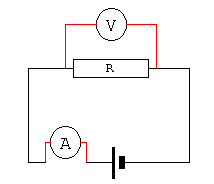Home > Electronics Tutorials > Direct Current (DC) Theory > Watts and Joules Tutorial

Direct Current (DC) Theory - Electronics Teaching

# Watts and Joules TutorialWhen current passes through a resistor, electrical energy is converted into heat. This heat is DISSIPATED into the surrounding air. The rate at which this dissipation occurs is called POWER and is measured in WATTS. The amount of power can be calculated by using one of three methods.

(1) Power = V x I watts
(2) Power = (V x V)/R watts
(3) power = (I x I)R watts
Also see the page with the VIRP wheel.

If we place a 10 ohm resistor across a 20 volt battery then Ohms law says that I = V/R = 20/2 =2 amps will flow.

Using (1)
power = 20 x 2 = 40 watts

Using (2)
power = (20 x 20)/10 = 400/10 = 40 watts.

using (3)
power = (2 x 2)x10 = 4 x 10 = 40 watts.

Using three different formulae we still arrive at the same answer.

We know that power is the rate at which energy is used. The amount of energy used is measured in JOULES.

Joules = watts x seconds, therefore watts = joules/seconds.

A 1000 watt fire will dissipate 1000 joules per second. With resistors, the greater the dissipation the hotter it gets, and the larger the resistor needs to be.

The electric meter in your house measures UNITS of electricity. A unit is when you use 1000 watts for one hour. This is called 1 Kilowatt hour.

It is kilowatts x hours. A 100 watt (0.1 kilowatts) lamp left on for 24 hours uses 0.1 x 24 = 2.4 units.

You or your parents are charged by the unit. If a unit cost 5 pence then the lamp would cost 12 pence a day to run. Switch it off and help to save the world.